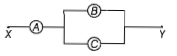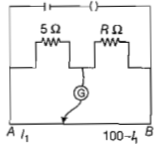The charge following through a resistance R varies with time  where a and b are positive constants. The total heat produced in R is

(a) $\frac{{a}^{3}R}{3b}$               (b) $\frac{{a}^{3}R}{2b}$

(c) $\frac{{a}^{3}R}{b}$               (d) $\frac{{a}^{3}R}{6b}$

Concept Questions :-

Heating effect of current
High Yielding Test Series + Question Bank - NEET 2020

Difficulty Level:

A potentiometer wire has a length 4 m and resistance 8Ω. The resistance that must be connected in series with the wire and an accumulator of emf 2V, so as to get a potential gradient 1mV per cm of the wire is

(a)32Ω

(b)40Ω

(c)44Ω

(d)48Ω

Concept Questions :-

Meter bridge and potentiometer
High Yielding Test Series + Question Bank - NEET 2020

Difficulty Level:

A, B, and C are voltmeters of resistance R, 1.5R, and 3R respectively as shown in the figure. When some potential difference is applied between X and Y, the voltmeter readings are VA, VB, and VC respectively. Then,1. VA=VB=VC

2. VA≠VB=VC

3. VA=VB≠VC

4. VA≠VB≠VC

Concept Questions :-

Combination of resistors
High Yielding Test Series + Question Bank - NEET 2020

Difficulty Level:

Across a metallic conductor of non-uniform cross-section, a constant potential difference is applied. The quantity which remains constant along the conductor is :

1. current density
2. current
3. drift velocity
4. electric field

Concept Questions :-

Current and current density
High Yielding Test Series + Question Bank - NEET 2020

Difficulty Level:

A potentiometer wire of length L and a resistance r are connected in series with a battery of e.m.f. ${E}_{o}$ and a resistance r1. An unknown e.m.f. is balanced at a length l of the potentiometer wire. The e.m.f. E will be given by

(a)$\frac{{E}_{o}rL}{\left({r}_{1}\right)l}$

(b)$\frac{{E}_{o}rl}{\left(r+{r}_{1}\right)L}$

(c)Eol/L

(d)$\frac{{E}_{o}rL}{\left(r+{r}_{1}\right)l}$

Concept Questions :-

Meter bridge and potentiometer
High Yielding Test Series + Question Bank - NEET 2020

Difficulty Level:

Two cities are 150 km apart. Electric power is sent from one city to another city through copper wires. The fall of potential per km is 8V and the  average resistance per km is 0.5 Ω. The power loss in the wire is

(a) 19.2W

(b) 19.2kW

(c) 19.2J

(d) 12.2kW

Concept Questions :-

Heating effect of current
High Yielding Test Series + Question Bank - NEET 2020

Difficulty Level:

The resistances in the two arms of the meter bridge are 5 and R , respectively. When the resistance R is shunted with an equal resistance, the new balance point is at 1.6${l}_{1}$. The resistance R, is(a)10Ω

(b)15Ω

(c)20Ω

(d)25Ω

High Yielding Test Series + Question Bank - NEET 2020

Difficulty Level:

A potentiometer circuit has been setup for finding the internal resistance of a given cell. The main battery, used across the potentiometer wire, has an emf of 2.0 V and a negligible internal resistance. The potentiometer wire itself is 4 m long. When the resistance, R, connected across the given cell, has values of

(i)infinity

(ii)9.5Ω

the 'balancing lengths', on the potentiometer wire are found to be 3m and 2.85m, respectively. The value of internal resistance of the cell is

(a) 0.25Ω

(b) 0.95Ω

(c) 0.5Ω

(d) 0.75Ω

Concept Questions :-

Meter bridge and potentiometer
High Yielding Test Series + Question Bank - NEET 2020

Difficulty Level:

A wire of resistance 4Ω is stretched to twice its original length. The resistance of a stretched wire would be :

(a)2Ω

(b)4Ω

(c)8Ω

(d)16Ω

Concept Questions :-

Combination of resistors
High Yielding Test Series + Question Bank - NEET 2020

Difficulty Level:

The internal resistance of a 2.1V cell which gives a current of 0.2A through a resistance of 10Ω is :

(a)0.2Ω

(b)0.5Ω

(c)0.8Ω

(d)1.0Ω

Concept Questions :-

Emf and terminal voltage